Skip to main content

# Modelling and simulation of flame cutting for steel plates with solid phases and melting

## Abstract

The goal of this work is to describe in detail a quasi-stationary state model which can be used to deeply understand the distribution of the heat in a steel plate and the changes in the solid phases of the steel and into liquid phase during the flame cutting process. We use a 3D-model similar to previous works from Thiébaud (J. Mater. Process. Technol. 214(2):304–310, 2014) and expand it to consider phases changes, in particular, austenite formation and melting of material. Experimental data is used to validate the model and study its capabilities. Parameters defining the shape of the volumetric heat source and the power density are calibrated to achieve good agreement with temperature measurements. Similarities and differences with other models from literature are discussed.

## Introduction

In industry, the flame cutting process occurs in the final stage of the processing of steel plates. By then, the desired properties of the steel have already been established with different heat treatment processes. Hence the goal is to perform the cutting process without altering the mechanical and chemical properties already settled. In the case of thermal cutting, a thin section is removed, named kerf, and a heat affected zone (HAZ) is created around the cutting edge of the remaining pieces, which can cause deviations to the expected properties of the steel owing to occurring solid-solid phase transitions in the HAZ, which will result in changes in the thermomechanical properties. Moreover, for certain process parameters it has been found that cold cracks can appear a couple of days after the cutting possibly due to tensile stress, hydrogen content and microstructural effects .

A deeper knowledge of the cutting process can help to identify the crucial factors to maintain the good quality of the steel plates. This can be done in a suitable and non-expensive way with the help of computer simulations after the physics of the problem has been understood. In contrast to welding, which is a similar physical process, there are very few publications studying thermal cutting from a modelling and numerical simulation point of view.

In Lindgren et al.  the authors use a 2D-model to study the temperature evolution in the section transverse to the direction of the flame cutting. The heat input is adjusted with experimental measurements of two isothermal lines. The paper reveals the decrease in residual stress with a pre-heating stage of the plate prior to cutting using a model for mechanical analysis. Jokiaho et al.  employ the commercial finite element software ABAQUS to model the flame cutting of thick plates. Again, a 2D-model is used to register the nodal temperature history in the transverse plane. Different values for the heat input are used for different cutting speeds. The aforementioned values are calibrated such that the temperatures in the middle plane of the plate (half the thickness) do not reach the melting point. The study focuses on the stress analysis under different operating conditions: thickness, speed, pre-heating stage. Bae et al.  work with ABAQUS for a 2D-model. The authors adopt a more complex definition of the heat flux. It is divided in three parts: upper surface due to the reaction between ethylene and oxygen, cutting line due to the reaction between oxygen and iron, and bottom of cutting line due to the heat conducted by the molten droplets. The model predicts the shape of the HAZ for diverse plate thicknesses, cutting speeds and flow rates of oxygen/ethylene. Thiébaud et al.  study the quasi-steady state of the temperature distribution during flame cutting with a 3D-model. The heat input is modelled as a volumetric heat source with a cylindrical-like shape. The simulation domain contains a gap related to an assumed kerf width. Two parameters are used to match the temperature profiles measured by several thermocouples on the top and bottom of the plate. These parameters are the heat density within the flame and the heat transfer coefficient in the aforementioned gap. Good matching with the experimental data is achieved.

In Gross , a sophisticated multi-physics 3D-model is considered including gas dynamics and the melting of steel. However, the author concludes that the computational effort for such a complex model is too costly for current hardware.

The present study is based on a quasi-stationary state 3D-model similar to the one used by Thiébaud. Using experimental data, the heat input power of the flame is adjusted to fit the data in the best possible way. The main novelty of the paper is the introduction of a quasi-stationary state (QSS) model comprising an energy balance coupled with two transport equations to model the occurring solid-liquid and solid-solid phase transitions. This approach allows for a more precise estimation of kerf and the heat affected zone.

The document is organized as follows. In Sect. 2, we describe a mathematical model for flame cutting of steel plates, consisting of a quasi-stationary heat equation coupled with a set of equations describing the phase transitions in steel. The considerations for numerical approximation and material data are presented in Sect. 3. Section 4 is devoted to the presentation of the results and comparison with experimental data used for validation followed by some concluding remarks in Sect. 5.

## Modelling

### Domain

Flame cutting of steel plates consists of removing a small amount of material from the plate along a straight cutting line dividing it in two pieces and leaving them with a very regular edge. For symmetry reasons, only one half of the plate will be considered, denoted by Ω̃. Due to the big size of the steel plate in comparison to the flame and the straight cutting trajectory, later on we will describe the cutting process by a quasi-stationary state (QSS) model. The plate moves with velocity v relative to the stationary torch. We define a cut-out Ω around the torch, which is represented by the subdomain Λ in Fig. 1. The symmetry plane is $$\{ y=0\}$$.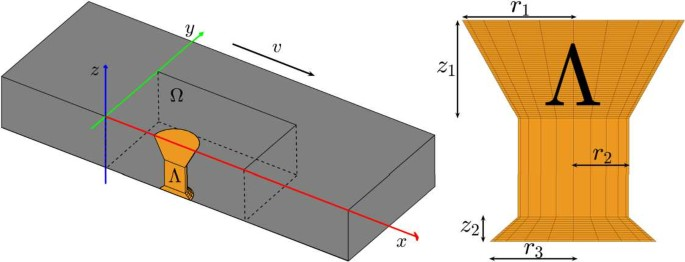Figure 1

### Heat source

The burning and removal of material is a complex process that can be described in three steps:

1. (i)

A torch located on top of the plate raises the steel to its ignition temperature ( 960°C ). For this, the torch creates a flame using a mixture of oxygen and a fuel gas, commonly acetylene, butane or propane.

2. (ii)

The iron in the steel reacts with the highly concentrated oxygen from the torch in an exothermic oxidation reaction adding more heat to the plate.

3. (iii)

The steel below the torch reaches melting temperature ( 1537°C). By heat conduction the melting temperature reaches the bottom of the plate. Immediately, the molten steel is flushed away by the high pressure oxygen stream.

These steps occur within a matter of seconds and additionally, the plate is moving in a fixed direction, thus separating the workpiece in two. The resultant edges of each new half of the steel are called cutting edges and the gap created is named kerf or groove. The process is ruled by two main factors: the cutting speed v and the flame. Depending on the steel properties and the thickness of the plate, a suitable speed must be chosen by the operators in the factory. The shape of the flame is determined by the torch used while the power depends on the combustion gas selected and the pressure of the oxygen and fuel gas stream.

Mathematically, the heat source $$Q(\boldsymbol {v})$$ can be characterized in terms of the shape of the flame created by the torch. It is defined as a constant power density [W/m3] within a particular volume Λ and assumed to be zero outside of it. More precisely, the power density is determined by a constant value for power P [W] divided by the volume of Λ. The heat source is uniformly distributed within the volume Λ, which is similar to a cylinder whose radius changes with height, specifically Λ can be identified as a cylinder with a truncated cone on the top and at the bottom (see Fig. 1). The variation of shape, and therefore heat, depending on height is based on physical reasons. While on the cutting plane most of the heat comes from the oxidation of iron and concentrates in a thin cylindrical volume below the torch, extra heat input exists close to the top and bottom surfaces of the plate: at the bottom by the presence of droplets of molten metal and on the top surface by the reaction between oxygen and fuel gas [1, 35].

In Fig. 2 two sets of isothermal curves in cross sections parallel and with growing distance to the cutting surface are depicted. The sets correspond to a speed of 135 and 270 mm/min, respectively, during the flame cutting process. The isothermal lines were estimated using the temperature records from thermocouples (attached to holes drilled in the plate), microstructure study and literature. Both sets start in the cutting edge of the remaining half after flame cutting. The cutting edge is almost aligned with the 1537°C curves. Its shape can be regarded as an experimental evidence for the assumed shape of the volume Λ. Moreover, higher temperatures are reached further within the plate in the slower case as the heat source has more influence and due to more time for heat conduction. This fact can also be seen in Jokiaho et al. , where a conclusion of the flame cutting experiments is that the heat affected zone width depends on cutting speed.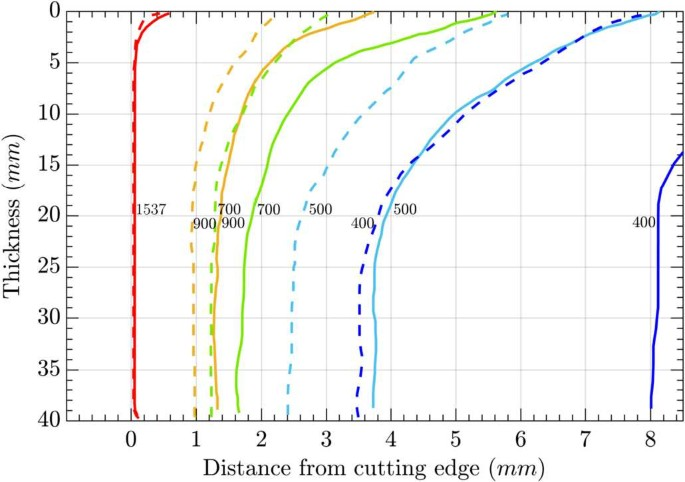Figure 2

Furthermore, it is important to mention that the kerf (removed area) in both cases is different, being smaller in the faster case. In Duan et al. , it is stated that the kerf width is generally assumed to be constant in most mathematical models of flame cutting. However, a large error may be produced if this variation in width with respect to thickness and cutting speed is neglected. Hence, we assume that the kerf size decreases with increasing speed and model this effect by changing the radius of Λ as a function of velocity v.

In view of all these considerations, we define Λ as

$$\varLambda(\boldsymbol {v})= \bigl\{ \boldsymbol {x} \in \widetilde {\varOmega }\vert (x-x_{\varLambda } )^{2}+ (y-y_{\varLambda} )^{2} \leq R(z,\boldsymbol {v})^{2}, -h\leq z \leq0 \bigr\} .$$
(1)

Here, $$(x_{\varLambda},y_{\varLambda})$$ are the coordinates of the symmetry axis of Λ. The simplest way to model a source with the above mentioned phenomena is with only five parameters that define the shape of the heat source along the complete thickness h of the plate: $$r_{1}$$, $$r_{2}$$, and $$r_{3}$$ are the upper, medium and lower radii, respectively, and $${z}_{1}$$ and $${z}_{2}$$ the heights of the top and bottom truncated cones, respectively (see Fig. 1).

We define the function $$R(z,\boldsymbol {v})$$ from Equation (1) reflecting the radial change of Λ according to height and speed in a separable way as a product of two functions, i.e.,

$$R(z,\boldsymbol {v})=C(\boldsymbol {v})\bar{R}(z),$$
(2)

with

$$\bar{R}(z)= \textstyle\begin{cases} \frac{r_{2}-r_{1}}{\bar{z}_{1}}z+r_{1}, & z>-\bar{z}_{1}, \\ r_{2}, & -\bar{z}_{1}\geq z\geq-h+\bar{z}_{2},\\ \frac{r_{2}-r_{3}}{\bar{z}_{2}} (z+h-\bar{z}_{2} )+r_{2}, & z< -h+\bar {z}_{2}. \end{cases}$$
(3)

The definition of $$\bar{R}(z)$$ manifest the difference in radii with height and can be seen in Fig. 3. On the other hand, $$C(\boldsymbol {v})$$ takes into account that the faster the plate moves the smaller the area of influence of the flame gets. Thus, for convenience, $$C(\boldsymbol {v})$$ is assumed to be a linear, monotonically decreasing function that causes the radii of Λ to be scaled with velocity.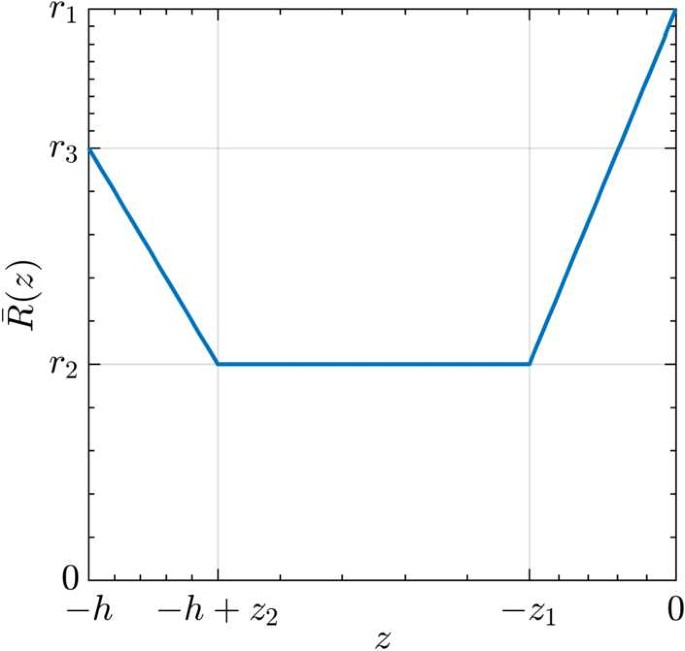Figure 3

All in all, the heat source $$Q(\boldsymbol {v})$$ is then defined as

$$Q(\boldsymbol {v})=\frac{P}{ \vert \varLambda(\boldsymbol {v}) \vert }\protect \raisebox {2pt}{\chi }_{\varLambda(\boldsymbol {v})}$$
(4)

with $$\protect \raisebox {2pt}{\chi }_{\varLambda(\boldsymbol {v})}$$ the characteristic function of the volume $$\varLambda(\boldsymbol {v})$$, i.e., it takes value 1 within $$\varLambda(\boldsymbol {v})$$ and 0 outside.

### Phase transitions

During a heating process, steel undergoes microstructural changes affecting its physical properties. At room temperature, combinations of four different phases can be distinguished in the steel: ferrite, pearlite, bainite and martensite. The presence and the proportion of each phase depends on the type of steel and its chemical composition, and on the heat treatment that the material has been subjected to. If the steel temperature grows sufficiently high, the previous solid phases are transformed into a new high temperature phase called austenite. This process is called austenitization. During a cooling cycle, this austenite is dissolved into the previous phases. The volume fraction of the remaining phases is determined by the local cooling rate. For more detailed information we refer to .

During flame cutting, around the cutting plane, a portion of steel is molten and removed while in the neighbourhood of this kerf a Heat Affected Zone (HAZ) is formed. This HAZ is generated at the cutting edge of the steel plate due to large thermal gradients . Phase transitions occur in the HAZ as the austenitization temperature is reached. The HAZ is an important region to study as it is the area where cracks have been found to appear, eventually leading to quality losses and customer complaints.

For modelling the flame cutting process it is important to account for the melting of steel and thus to estimate the size of the kerf. To this end, we add the relative fraction of molten steel as an extra phase to our phase transition model. In Hömberg et al. , a transient model based on the Leblond–Devaux model is proposed that reproduces the relative volume fractions of the different solid phases of steel during a heating and cooling cycle.

We define the HAZ as the area where austenitization has happened. Thus, to predict its size, which will provide information about the possible occurrence of cracks, it is sufficient to keep track of the formation of austenite. With this in consideration, we propose a reduced model, based on , consisting of a rate law for austenite a and liquid phase (molten steel) l with θ being the temperature. The corresponding system of equations for these two phases is the following:

\begin{aligned}& \dot{l} = \frac {1}{\tau _{l}} \bigl[l_{\mathrm{eq}}(\theta ) - l\bigr]_{+}, \end{aligned}
(5)
\begin{aligned}& \dot{a} = \frac {1}{\tau _{a}} \bigl[a_{\mathrm{eq}}(\theta ) - a - l\bigr]_{+}-\dot{l}, \end{aligned}
(6)
\begin{aligned}& l(0)=a(0)=0. \end{aligned}
(7)

Here, $$l_{\mathrm{eq}}$$ and $$a_{\mathrm{eq}}$$ represent the equilibrium volume fraction of liquid and austenite phase, respectively, at temperature θ. The equilibrium volume fraction of the liquid phase $$l_{\mathrm{eq}}(\theta)$$ should have the maximum value 1, when the temperature exceeds 1537°C (melting point). Tacitly neglecting a mushy zone we define $$l_{\mathrm{eq}}(\theta)=H(\theta-1537)$$, where H is the Heaviside function. In addition, we define the positive part function as $$[x ]_{+}=xH(x)$$.

For the austenite phase a, equation (6) reflects the fact that no austenite should form at the expense of liquid during heating but austenite can be transformed to liquid phase. Here, $$a_{\mathrm{eq}}(\theta)$$ is the austenite equilibrium volume fraction and can be derived from a non-isothermal austenitization diagram. It has value 0 below austenitization temperature $$A_{s}$$ and then is assumed to increase linearly until the maximum value 1 is reached at $$A_{f}$$ temperature, both depending on the chemical composition of the steel grade under consideration. There are two time constants $$\tau_{l}$$ and $$\tau_{a}$$ in equations (5) and (6) to adjust the transformation kinetics. The necessary initial conditions for liquid and austenite are stated in (7).

Figure 4 shows the evolution of phase volume fractions obtained after solving (5)–(7) with a temperature history $$\theta(t)$$ from flame cutting. In a small fraction of time, the rapid rise in temperature takes the steel to austenitization temperature and immediately into melting.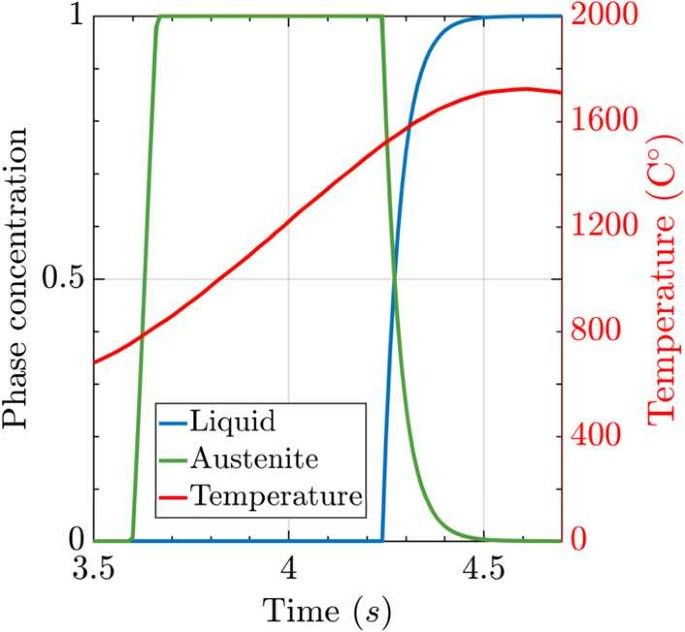Figure 4

This model can be summed up as a system of rate laws (ODEs) determined by temperature θ and the already present phase fractions,

$$\dot{\boldsymbol {\zeta }}(t) = \boldsymbol {f}(\theta, \boldsymbol {\zeta }),$$
(8)

where ζ is the vector of phase fractions. For the liquid and austenite model we have

$$\boldsymbol {\zeta }= ( l, a )^{T} \quad\text{and} \quad \boldsymbol {f}= \bigl( f_{l}(\theta ,l), f_{a}(\theta,a,l) \bigr)^{T}.$$
(9)

Due to the big size of the steel plate in comparison to the flame and the straight cutting trajectory, we now switch to a quasi-stationary state (QSS) setting to model the flame cutting heating process, allowing for more efficient simulation. The requirement for a valid QSS approach is that an observer located on the heat source, i.e., the flame, will notice no change in the temperature distribution around it, while the plate moves with constant velocity under the torch. To obtain the QSS equation for the phase evolution system, first we consider the equation to be defined in the whole spatial domain Ω̃,

$$\dot{\boldsymbol {\zeta }}(\boldsymbol {x},t) = \boldsymbol {f}(\theta, \boldsymbol {\zeta }), \quad\forall \boldsymbol {x}\in \widetilde {\varOmega }$$
(10)

We describe the moving plate relative to the stationary heat source . To this end, we replace x by a new coordinate ξ subject to a given constant cutting speed v, i.e., we define

$$\boldsymbol {\xi}= \boldsymbol {x} - \boldsymbol {v}t$$
(11)

such that the phase fractions $$\zeta_{i}$$ are stationary, i.e., we assume

$$0= \frac{d}{d t} \zeta_{i}(\boldsymbol {x} - \boldsymbol {v}t,t)=-\boldsymbol {v}\cdot\nabla \zeta_{i}+\frac {\partial\zeta_{i}}{\partial t}.$$

Then, each component of Equation (10) can be rewritten as

$$\boldsymbol {v}\cdot\nabla\zeta_{i} = f_{i}( \theta, \boldsymbol {\zeta }), \quad\forall \boldsymbol {\xi}\in \varOmega.$$
(12)

The domain Ω is stationary and has to be chosen sufficiently big.

Equation (12) is also known as transport equation. Altogether, the time-dependent evolution model of liquid and austenite phase (5)–(7) can be rewritten as the following quasi-stationary system of transport equations,

\begin{aligned}& \boldsymbol {v}\cdot\nabla l= \frac {1}{\tau _{l}} \bigl[l_{\mathrm{eq}}(\theta ) - l\bigr]_{+}, \quad \text{in } \varOmega, \end{aligned}
(13)
\begin{aligned}& \boldsymbol {v}\cdot\nabla a= \frac {1}{\tau _{a}} \bigl[a_{\mathrm{eq}}(\theta ) - a - l\bigr]_{+}-\boldsymbol {v}\cdot\nabla l,\quad \text{in } \varOmega, \end{aligned}
(14)
\begin{aligned}& l=a=0, \quad \text{on } \{x=0\} , \end{aligned}
(15)
\begin{aligned}& \frac {\partial l}{\partial \boldsymbol {n}}=\frac {\partial a}{\partial \boldsymbol {n}}=0, \quad \text{on } \partial\varOmega \setminus\{x=0\}. \end{aligned}
(16)

The system of equations (13)–(16) reflects the development of the liquid and austenite phase during a heating process while taking into account the movement of the domain Ω with respect to the heat source Λ. From now on, for notation purposes, we will denote the variable ξ as x and drop the t dependency. It has to be noticed that the previous initial conditions from (7) are substituted by boundary conditions. Equation (15) is a Dirichlet condition indicating that there is no volume fraction of liquid or austenite in the in-flow plane, far from the heat source. Otherwise, the homogeneous Neumann condition (16) reflects that stationarity has been achieved on the outflow boundary.

### Complete system

The goal of the model is to determine the heat distribution in a moving steel plate during flame cutting with the subsequent HAZ and the trail of molten steel. The big dimensions of a steel plate (400 mm long) in comparison to the heat affected zone caused by the flame (5 mm) allow to consider that a quasi-stationary state (QSS) is reached in the workpiece. Accordingly, for the temperature θ in the plate, we can employ the quasi-stationary state (QSS) heat equation ,

$$\rho (\theta ) C_{p} (\theta ) (\boldsymbol {v}\cdot\nabla \theta ) -\nabla\cdot \bigl(\kappa (\theta, l ) \nabla\theta \bigr)=q \quad\text{in } \varOmega.$$
(17)

Different material parameters are required for this equation: density ρ, specific heat capacity $$C_{p}$$ and heat conductivity $$\kappa_{0}$$. One important aspect is that these properties are temperature dependent, adding non-linearities to equation (17).

As mentioned earlier, the molten steel is flushed away during the cutting process. This has to be taken into account, otherwise, the liquid layer would act as an additional heat source (see ) increasing the HAZ artificially. To incorporate this effect into our model we propose a mixture ansatz for the heat conductivity, i.e.

$$\kappa (\theta, l ) = \kappa_{0}(\theta) (1-l) + \delta\kappa _{0}(\theta) l.$$
(18)

Here, $$\delta>0$$ is a small parameter chosen such that on the one hand heat diffusion through the kerf is negligible and on the other hand numerical instabilities caused by a degenerating diffusion coefficient are avoided.

The term q on the right hand side of (17) is related to the heat source and therefore, to the flame and the iron burning exothermic reaction. Furthermore, this term should also include the heat absorbed and released during phase transitions, known as latent heat. For simplicity, we will restrain the model to consider only liquid and austenite phases (13)–(16), then we can write the heat source as

$$q = Q(\boldsymbol {v}) - \rho (\theta ) L_{l} f_{l}(\theta,l) - \rho (\theta ) L_{a} f_{a}(\theta,a,l).$$
(19)

The definition of q includes the term $$Q(\boldsymbol {v})$$ described already in Sect. 2.2. The velocity v is a vector which, in this case, has only x as non-zero component as the trajectory of the plate is a straight line along the x axis: $$\boldsymbol {v}=(v,0,0)^{T}$$. The heat absorbed by the phase transformations is determined by the steel density $$\rho(\theta)$$ and the latent heat $$L_{i}$$ and function $$f_{i}$$ of each phase. The functions $$f_{i}$$ are specified in equations (13) and (14).

Following Sect. 2.3, we add the QSS phase equations for austenite and liquid as they are coupled with the heat equation due to being temperature dependant. These equations with adequate boundary conditions comprise the system of equations (20)–(30). Considering $$\theta_{a}$$ as the ambient temperature, Newton’s cooling law is imposed on the top and bottom of the plate (23)–(24) to describe the exchange of heat with surrounding air with the convection factors $$h_{1}$$ and $$h_{2}$$. The vector n is an outward normal unit vector to the corresponding surface. The temperature of the plate before the process is $$\theta_{a}$$ and far enough from the torch, the temperature suffers no change. Therefore, a Dirichlet condition is enforced on the plane $$\{y=y_{\mathrm{max}}\}$$ and on the inflow plane $$\{x=0\}$$, see equations (25)–(27). Finally, on the symmetry plane $$\{y=0\}$$ and on the outflow boundary $$\{ x=x_{\mathrm{max}}\}$$, a homogeneous Neumann condition is imposed implicating that there is no heat flux in the normal direction. The values of $$x_{\mathrm{max}}$$ and $$y_{\mathrm{max}}$$ must be chosen sufficiently big, such the influence on the temperature and phase fractions from the artificial boundary conditions is negligible. The boundary conditions for the phase fractions have already been explained above.

\begin{aligned}& \rho (\theta ) C_{p} (\theta ) (\boldsymbol {v}\cdot\nabla\theta ) -\nabla \cdot \bigl(\kappa (\theta, l ) \nabla\theta \bigr) = q, \quad\text{in } \varOmega, \end{aligned}
(20)
\begin{aligned}& \boldsymbol {v}\cdot\nabla l= f_{l}(\theta,l), \quad\text{in } \varOmega, \end{aligned}
(21)
\begin{aligned}& \boldsymbol {v}\cdot\nabla a= f_{a}(\theta,a,l), \quad\text{in } \varOmega, \end{aligned}
(22)
\begin{aligned}& -\kappa(\theta, l)\frac {\partial \theta}{\partial \boldsymbol {n}}=h_{1}(\theta- \theta_{a}), \quad\text{on } \{z=0\}, \end{aligned}
(23)
\begin{aligned}& -\kappa(\theta, l)\frac {\partial \theta}{\partial \boldsymbol {n}}=h_{2}(\theta- \theta_{a}), \quad\text{on } \{z=z_{\mathrm{max}}\}, \end{aligned}
(24)
\begin{aligned}& \theta=\theta_{a}, \quad\text{on } \{x=0\}, \end{aligned}
(25)
\begin{aligned}& \frac {\partial \theta}{\partial \boldsymbol {n}}=0, \quad\text{on } \{x=x_{\mathrm{max}}\}, \end{aligned}
(26)
\begin{aligned}& \theta=\theta_{a}, \quad\text{on } \{y=y_{\mathrm{max}} \}, \end{aligned}
(27)
\begin{aligned}& \frac {\partial \theta}{\partial \boldsymbol {n}}=0, \quad\text{on } \{y=0\}, \end{aligned}
(28)
\begin{aligned}& l=a=0, \quad\text{on } \{x=0\}, \end{aligned}
(29)
\begin{aligned}& \frac {\partial l}{\partial \boldsymbol {n}}=\frac {\partial a}{\partial \boldsymbol {n}}=0, \quad\text{on } \partial\varOmega \setminus\{x=0\}. \end{aligned}
(30)

## Numerical approach

The purpose of the model presented is to predict the temperature distribution, the HAZ size in terms of the fraction of austenite and the kerf, i.e., the trail of molten steel produced by flame cutting of a high strength steel plate with 40 mm thickness. We apply the Finite Element Method (FEM) to solve the system (20)–(30) and the requirements for this are detailed in the following.

The material properties required for the model are the density, specific heat capacity and thermal conductivity of the considered Raex® 400 steel grade. These temperature dependant properties were obtained using the commercial software JMatPro® , which mainly requires as input the chemical composition of the steel (Table 1).

After defining the geometric parameters and constants of the model, it is possible to solve the system of equations (20)–(30). This is achieved using the finite element package pdelib2 developed and maintained at WIAS. The non-linearities together with the coupling were solved using a fixed-point algorithm and an adaptive mesh module was used to refine the mesh based on a residual error estimator resolving regions with steep gradients for the temperature and phase fractions .

As mentioned before, the heat loss due to vanishing heat conductivity in the kerf is modelled by the mixture ansatz described in (18). We found the choice $$\delta=0.01$$ a good compromise yielding both a numerically stable scheme and vanishing heat transport in the kerf.

Note that the phase transition model (21)–(22) represents a system of transport equations and cannot be solved directly using FEM in 3D without encountering erroneous results . The first step taken to deal with this problem is to add artificial diffusivity to the equation. This consists in adding a diffusion term with a coefficient $$\varepsilon>0$$.

$$\boldsymbol {v}\cdot\nabla \boldsymbol {\zeta }- \varepsilon\Delta \boldsymbol {\zeta }=f(\theta, \boldsymbol {\zeta }).$$
(31)

The value of ε must be small enough to avoid too much diffusion but big enough to be effective. For our simulations, we found $$\varepsilon=10^{-8}\mbox{ m}^{2}\mbox{/s}$$ to produce an adequate outcome, but still a further step is required. Equation (31) is a steady-state linear scalar convection-diffusion equation with a convection-dominated regime. It is well known that, with this regime, the solutions from FEM contain spurious oscillations. A stabilization method is required. We chose the Streamline-Upwind Petrov–Galerkin (SUPG) method that adds diffusion in the direction of the streamlines .

In order to highlight the need for the aforementioned steps to solve appropriately equations (21)–(22) when using FEM in a 3D domain, Fig. 5 represent the numerical results for the liquid phase in each mentioned step. A temperature distribution in a plate was retrieved after solving the heat equation with a flame cutting. This temperature field was used as input to solve directly the transport equation (21) leading to erratic results (see figure on the left). Then, the result obtained with the addition of artificial diffusivity (31) is in the middle of Fig. 5 while the figure on the right displays the final result with the introduction of the SUPG method.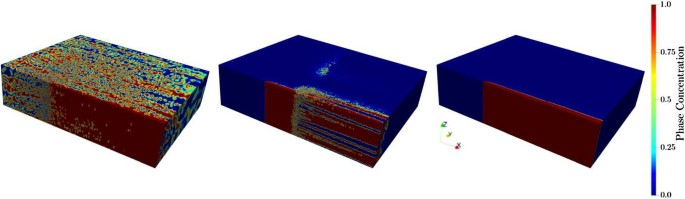Figure 5

## Results and validation

Experimental data from two experiments are used to calibrate the volumetric heat source. Both experiments consider a 40 mm Raex® 400 steel plate but different cutting speed: 135 and 270 mm/min. The results comprise isothermal curves in different planes transverse to the cutting direction, see Fig. 2. In this section, the main results presented belong to the 135 mm/min case as it was used as reference case. Furthermore, the results obtained for 135, 202.5 and 270 mm/min cutting speeds are compared.

From the experimental data, the shape of the 1537°C isothermal line in Fig. 2 plus the estimated size of the kerf of 1 mm for the case with a cutting speed of 135 mm/min suggested to use the same values for the volumetric heat source as Thiébaud . These values are $$r_{1}= 2\mbox{ mm}$$, $$r_{2}=r_{3}= 1\mbox{ mm}$$ and $${z}_{1}={z}_{2}= 2\mbox{ mm}$$. The power P within Λ was set to 7.35 kW. The size of the computational domain was established to be in accordance with the Dirichlet conditions: $$y_{\mathrm{max}}= 100\mbox{ mm}$$ and $$x_{\mathrm{max}}= 140\mbox{ mm}$$. The vertical axis of the heat source Λ was located at $$(40\mbox{ mm}, 0)$$. Heat transfer coefficients $$h_{1}$$ and $$h_{2}$$ were set to 5 W/(m2K) and the external temperature $$\theta_{a}$$ was 25°C. Regarding the phase equations, the latent heat values were 272 and 16 kJ/kg for liquid and austenite, respectively. The values of $$\tau_{l}$$ and $$\tau_{a}$$ representing the transformation speed were 1E-2 and 5E-1 s. It was found that is important that $$\tau_{l}$$ is smaller than $$\tau_{a}$$ to produce a small interphase region.

### Case 135 mm/min

The main focus was on this case as it was used as the reference case. A general view of the temperature distribution around the heat source in the remaining steel is given in Fig. 6. In this figure, we depict the temperature field in the computational domain where no liquid phase is present, i.e. without the kerf. The features of the heat source Λ can be sensed from the isothermal surfaces with the highest temperature values. As expected, the maximum temperature in the remaining plate is below the melting point (1537°C).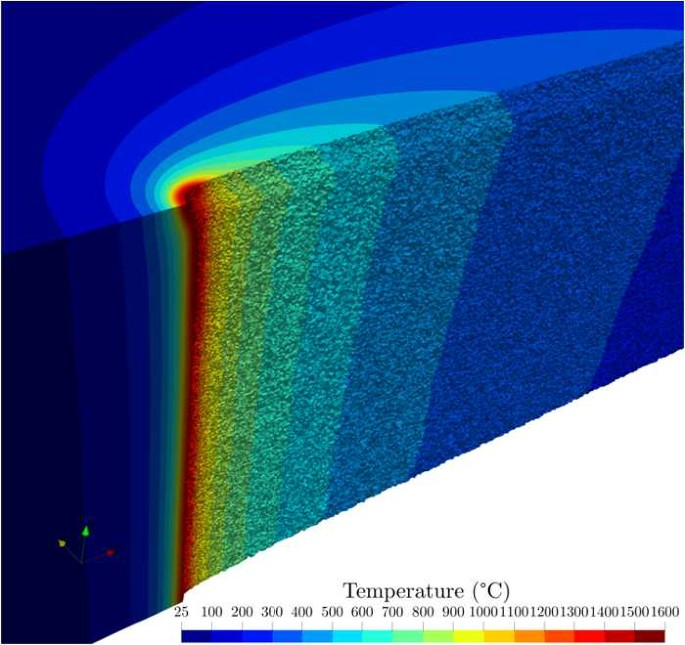Figure 6

The corresponding trail of liquid phase (molten steel) and austenite phase produced with the heat source is shown in Fig. 7. Both phases start approximately at the isothermal surface corresponding to the temperature where the equilibrium volume fraction of each phase is 1, then the phases are transported in the direction of the movement, the x-direction. The trail of liquid is the closest to the cutting plane as expected from the higher temperatures being in this plane. In Fig. 7, there is a transparent slice of the liquid and austenite volumes such that the inner profile of both phases along the thickness of the plate can be seen. The liquid phase is wider on top while in the middle and bottom it remains the same. On the other hand, the width of austenite is narrower on top while constant along the rest of the thickness.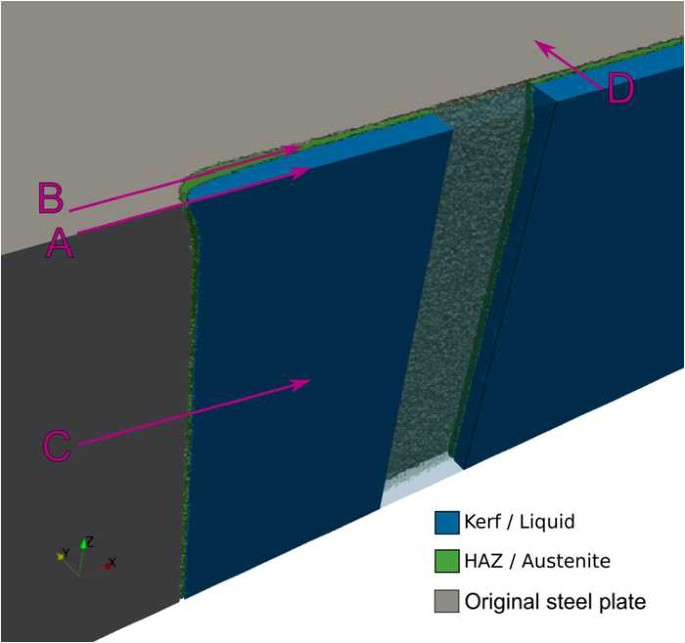Figure 7

To understand more clearly the next results, Fig. 7 also illustrates the location of the straight lines A, B, C and D along which the results where extracted. In Fig. 8, we can see the phases evolution along lines A (left) and B (right). As A crosses a zone of rapid increase in temperature the austenite phase grows very quickly until the melting point is reached and it decreases while the liquid phase grows. On the other hand, the B line is far enough from the torch and the temperature only causes austenite growth.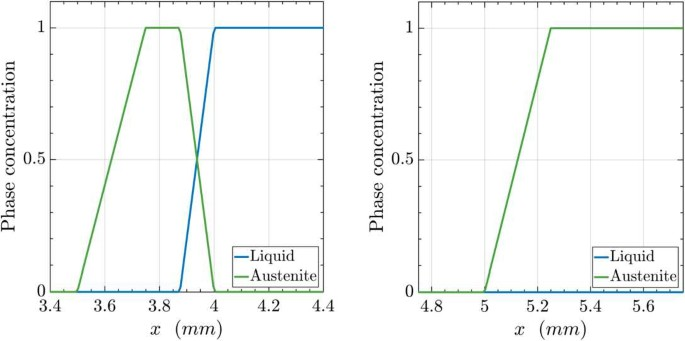Figure 8

As the phases can be identified with the kerf and the Heat Affected Zone (HAZ), respectively, we can retrieve the width of both areas with line D. This is presented in Fig. 9. On the transverse plane to the cutting direction we find the liquid phase, then a very small two-phase region and finally the remaining plate with the austenitized and the non-affected area. On the left-hand side the result is shown with the heat losses due to vanishing heat diffusion in the kerf. The right-hand side depicts the result with heat diffusion in the kerf (i.e., $$\delta=1$$ in (18)) leading to a wider HAZ as expected. A further comparison of kerf and HAZ cross-section in the case with (left) and without heat losses in the kerf (right) is shown in Fig. 10.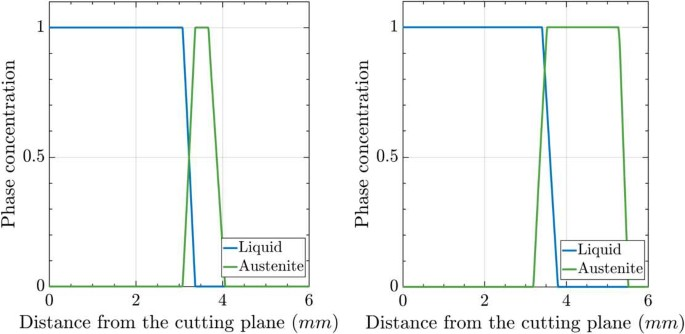Figure 9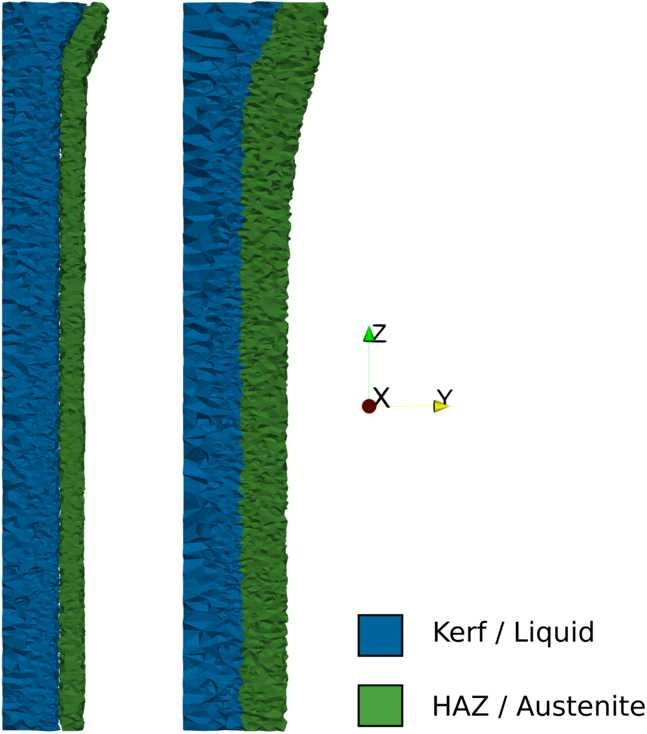Figure 10

In the remaining plate, a rapid increase in temperature and the cooling afterwards can be observed in Fig. 11. We track the temperature on the top (left) and middle (right) of the plate using lines A and C. Moreover, these lines are shifted in the y-direction to obtain more information about temperatures reached in the remaining plate. As shown in Fig. 6, the maximum temperature is reached on the top of the plate. The curves closest to the cutting line vanish as the position corresponds to the liquid phase region.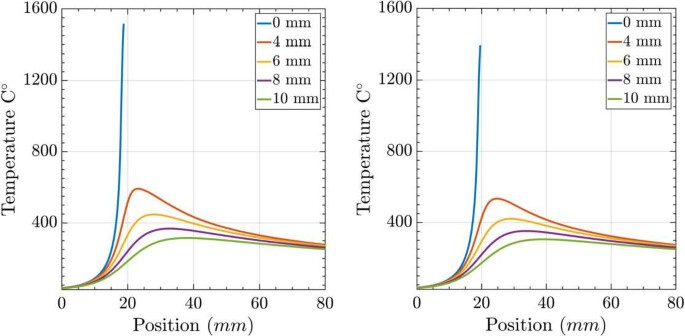Figure 11

### Case 202.5 and 270 mm/min

To see the effect of different cutting speeds we multiplied the base speed 135 mm/min, by a factor 1.5 and 2, resulting in 202.5 and 270 mm/min. As it is explained in the model, the velocity v affects the volume Λ where the heat source is defined. Specifically the speed affects the value of $$C(\boldsymbol {v})$$ which scales the radii of Λ with speed (cf. (2)). The function $$C(\boldsymbol {v})$$ is assumed to be linear and monotonically decreasing. More precisely, for the base case, we choose $$C(135)=1$$, while $$C(202.5)=0.75$$ and $$C(270)=0.5$$.

The features of the results obtained with faster velocities are the same qualitatively. Quantitatively, with higher speed, the influence area of the torch and the temperature distribution produce a smaller kerf and HAZ. In Table 2, the average values of kerf and HAZ width for the three cases are shown. Table 2 provides a good insight of the trends: smaller region width with increasing speed and greater values for the kerf on the top than in the middle and at the bottom of the plate.

As the kerf width is known after the numerical simulation, we can focus on the remaining plate. In Fig. 12, the temperature fields in the transverse plane to the cutting direction for the three cases are aligned to the resulting cutting edge. Thus, we can observe the difference in the temperature reached within the plate. As expected, with slower speed higher temperatures reach further into the plate.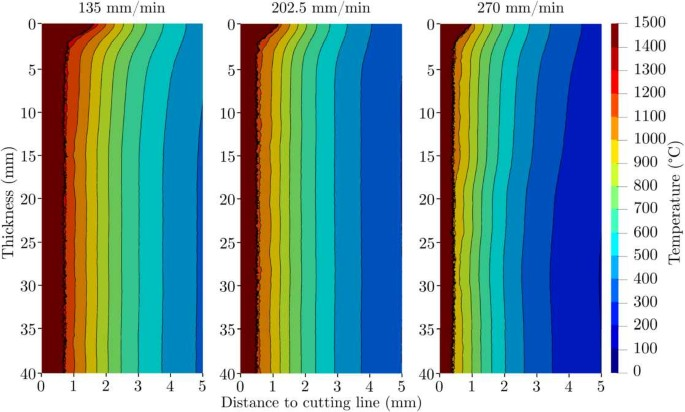Figure 12

## Discussion of results and conclusions

The model developed provides relevant information about the temperature distribution along the steel plate during the flame cutting process. The validated results can be used as a temperature history in every coordinate of the plate, allowing further studies involving solid phase changes or residual stresses. In general there is a good correspondence between the temperature values from experimental experience and those obtained after numerical simulation.

Two different approaches are possible for consideration of removal of material during the simulation [4, 5], either the domain starts in the cutting plane (torch position) or in the cutting edge (resultant edge after cutting). In Thiébaud  a mix of both options is used as the domain includes a predefined kerf for the area already affected by the flame. In this work, the domain starts in the cutting plane allowing us to describe the kerf size as the liquid phase created during flame cutting instead of predefining it.

The heat losses occurring in the kerf were identified as noteworthy in . Thus, we consider them by reducing the heat conductivity in the liquid region. We are convinced that this approach helps achieving more realistic values in the remaining plate.

The disagreement between numerical results and experimental data is higher in the surface area (compare Figs. 2 and 12). More heat should be spread in the y-direction. In practice, this effect is caused by a deflection of the flame on top of the plate. A more complex definition of the heat source in Bae et. al.  or  may provide more realistic results. For example in , it is divided into three terms, one of them is a Gaussian function which is only defined in the upper surface of the plate and may help balancing the temperatures obtained numerically, producing less heat in the cutting line but more into the width of the plate as should be expected according to experimental data.

Thiébaud and Jokiaho [1, 4] get similar temperature profiles to the ones displayed in Fig. 11. The total power required by Thiébaud is 18.8 kW, which is higher than the one estimated in this study, 7.35 kW. This difference is mostly related to the different treatment of the kerf as explained before.

The inclusion of quasi-stationary phases transition models for liquid and austenite in the present paper provides a new tool for a deeper understanding of the flame cutting process. The liquid phase fraction allows for a rather precise description of the kerf while the austenite fraction does the same for the heat affected zone. The trend found of decreasing width of the HAZ with increasing speed (Table 2) is also observed in experimental results in Jokiaho et al. . In their experiments the HAZ is assumed to be formed by a martensitic region, a two phase region and a tempered region. The width of the HAZ on the top of the plate is thinner than in the rest of the plate. This feature is inconsistent with experimental results [1, 5]. The reason for this is a thickening of the kerf close to the surface and accordingly less heat to create a wider HAZ close to the surface. However, this could be changed by changing the heat source which has been defined to match data from a different source.

All in all, it is fair to conclude that the model derived in the present paper covers all essential features of the flame cutting process of steel. For more reliable quantitative predictions a more precise adjustment of phenomenological model parameters based on refined measurements will be necessary. In a forthcoming paper, the optimal control of the pre-heating for the flame cutting process will be discussed in order to mitigate the danger of cold cracks.

## Abbreviations

HAZ:

Heat Affected Zone

QSS:

Quasi-stationary state

ODE:

Ordinary Differential Equation

FEM:

Finite Element Method

SUPG:

Streamline-Upwind Petrov–Galerkin stabilization method

WIAS:

Weierstrass Institute for Applied Analysis and Stochastics

## References

1. 1.

Thiébaud R, Drezet JM, Lebet JP. Experimental and numerical characterisation of heat flow during flame cutting of thick steel plates. J Mater Process Technol. 2014;214(2):304–10. https://doi.org/10.1016/j.jmatprotec.2013.09.016.

2. 2.

SSAB AB. RAEX welding and thermal cutting. 2020. https://ssabwebsitecdn.azureedge.net/-/media/files/en/raex/3003-raex-welding-and-thermal-cutting-2020.pdf. Accessed 15 Feb 2020.

3. 3.

Lindgren LE, Carlestam A, Jonsson M. Computational model of flame-cutting. J Eng Mater Technol. 1993;115(4):440–5. https://doi.org/10.1115/1.2904243.

4. 4.

Jokiaho T, Laitinen A, Santa-aho S, Isakov M, Peura P, Saarinen T, et al.. Characterization of flame cut heavy steel: modeling of temperature history and residual stress formation. Metall Mater Trans B. 2017;48(6):2891–901. https://doi.org/10.1007/s11663-017-1090-x.

5. 5.

Bae KY, Yang YS, Yi MS, Park CW. Numerical analysis of heat flow in oxy-ethylene flame cutting of steel plate. Proc Inst Mech Eng, B J Eng Manuf. 2018;232(4):742–51. https://doi.org/10.1177/0954405416654183.

6. 6.

Gross MS. On gas dynamic effects in the modelling of laser cutting processes. Appl Math Model. 2006;30(4):307–18. https://doi.org/10.1016/j.apm.2005.03.021.

7. 7.

Bolobov VI. Conditions for ignition of iron and carbon steel in oxygen. Combust Explos Shock Waves. 2001;37:292–6. https://doi.org/10.1023/A:1017523922778.

8. 8.

Jokiaho T, Santa-aho S, Järvinen H, Honkanen M, Peura P, Vippola M. Effect of microstructural characteristics of thick steel plates on residual stress formation and cracking during flame cutting. Mater Perform Charact. 2018;7(4):655–74. https://doi.org/10.1520/MPC20170083.

9. 9.

Duan J, Man HC, Yue TM. Modelling the laser fusion cutting process: I. Mathematical modelling of the cut kerf geometry for laser fusion cutting of thick metal. J Phys D, Appl Phys. 2001;34(14):2127–34. https://doi.org/10.1088/0022-3727/34/14/308.

10. 10.

Callister WD, Rethwisch DG. In: Materials science and engineering: an introduction. Hoboken: Wiley; 2007. p. 290–303.

11. 11.

Hömberg D, Weiss W. PID control of laser surface hardening of steel. IEEE Trans Control Syst Technol. 2006;14(5):896–904. https://doi.org/10.1109/TCST.2006.879978.

12. 12.

Rosenthal D. Mathematical theory of heat distribution during welding and cutting. Weld J. 1941;20:220–34.

13. 13.

Saunders N, Guo UKZ, Li X, Miodownik AP, Schillé JP. Using JMatPro to model materials properties and behavior. JOM. 2003;55(12):60–5. https://doi.org/10.1007/s11837-003-0013-2.

14. 14.

Hömberg D, Liu Q, Montalvo-Urquizo J, Nadolski D, Petzold T, Schmidt A, et al.. Simulation of multi-frequency-induction-hardening including phase transitions and mechanical effects. Finite Elem Anal Des. 2016;121:86–100. https://doi.org/10.1016/j.finel.2016.07.012.

15. 15.

Augustin M, Caiazzo A, Fiebach A, Fuhrmann J, John V, Linke A, et al.. An assessment of discretizations for convection-dominated convection–diffusion equations. Comput Methods Appl Mech Eng. 2011;200(47):3395–409. https://doi.org/10.1016/j.cma.2011.08.012.

Download references

### Acknowledgements

The authors want to thank SSAB AB for providing experimental data.

Not applicable.

## Funding

The authors are grateful for the support by the European Union’s Horizon 2020 research and innovation programme under the Marie Skłodowska-Curie grant agreement No. 675715 (MIMESIS).

## Author information

Authors

### Contributions

MJA developed the mathematical model and simulation tool with support from DH, RL and TP. PM provided data, information and technical view of the flame cutting industrial process. All authors contributed to the discussion of results and the manuscript text. All authors read and approved the final manuscript.

### Corresponding author

Correspondence to Manuel J. Arenas.

## Ethics declarations

### Competing interests

The authors declare that they have no competing interests.

## Rights and permissions

Open Access This article is licensed under a Creative Commons Attribution 4.0 International License, which permits use, sharing, adaptation, distribution and reproduction in any medium or format, as long as you give appropriate credit to the original author(s) and the source, provide a link to the Creative Commons licence, and indicate if changes were made. The images or other third party material in this article are included in the article’s Creative Commons licence, unless indicated otherwise in a credit line to the material. If material is not included in the article’s Creative Commons licence and your intended use is not permitted by statutory regulation or exceeds the permitted use, you will need to obtain permission directly from the copyright holder. To view a copy of this licence, visit http://creativecommons.org/licenses/by/4.0/.

Reprints and Permissions